1. /
2. CBSE
3. /
4. Class 10
5. /
6. Mathematics
7. /
8. CBSE Question Paper 2011...

# CBSE Question Paper 2011 class 10 Mathematics### myCBSEguide App

Download the app to get CBSE Sample Papers 2023-24, NCERT Solutions (Revised), Most Important Questions, Previous Year Question Bank, Mock Tests, and Detailed Notes.

CBSE Question Paper 2011 class 10 Mathematics conducted by Central Board of Secondary Education, New Delhi in the month of March 2011. CBSE previous year question papers with solution are available in myCBSEguide mobile app and cbse guide website. The Best CBSE App for students and teachers is myCBSEguide which provides complete study material and practice papers to cbse schools in India and abroad.

Question Paper 2011 class 10 Mathematics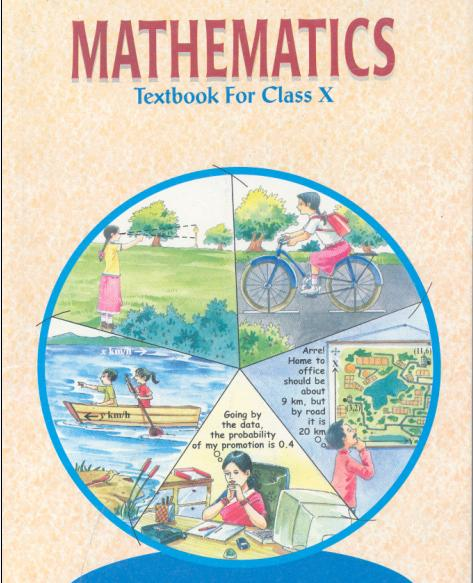## Class 10 Mathematics list of Chapters

1. Real Number
2. Polynomials
3. Pair of Linear Equations in Two Variables
5. Arithmetic Progressions
6. Triangles
7. Coordinate Geometry
8. Introduction to Trigonometry and its Applications
9. Circles
10. Constructions
11. Area Related to Circles
12. Surface Area and Volumes
13. Statistics and Probability

## Last Year Question Paper Class 10 Mathematics 2011

General Instructions:

(i) All questions compulsory.
(ii) The question paper consists of 34 questions divided into four sections – A, B, C, and D.
(iii) Section A contains 10 questions of 1 mark each, which are multiple choice type questions, Section B contains 8 questions of 2 marks each, Section C contains 10 questions of 3 each and Section D contains 6 questions of 4 marks each.
(iv) There is no overall choice in the paper. However, internal choice is provided in one questions of 2 marks, three questions of 3 marks and two questions of 4 marks.
(v) Use of calculators is not permitted.

### Section A

Question number 1 to 10 carry 1 mark each. For each of the question number 1 to 10, four alternative choice have been provided, of which only one is correct. Select the correct choice.

1. The roots of the equation x2 – 3x – m(m+3)=0, where m is a constant, are
(A) m, m + 3
(B) – m, m + 3
(C) m, – (m + 3)
(D) – m, – (m + 3)

2. If the common difference of A.P. is 3, then a20 – a15 is
(A) 5
(B) 3
(C) 15
(D) 20

3. In Figure 1, O is the centre of a circle, PQ is a chord and PT is the tangent at P. IfthenTPQ is equal to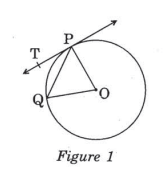(A) 55°
(B) 70°
(C) 45°
(D) 3

4. In Figure 2, AB and AC are tangents to the circle with centre O such thatThenBOC is equal to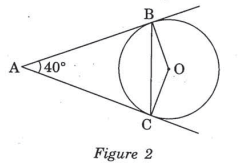(A) 40°
(B) 50°
(C) 140°
(D) 150°

5. The perimeter (in cm) of a square circumscribing a circle of radius a cm, is
(A) 8 a
(B) 4 a
(C) 2 a
(D) 16 a

6. The radius (in cm) of the largest right circular cone that can be cut out from a edge 4.2 cm is
(A) 4.2
(B) 2.1
(C) 8.4
(D) 1.05

7. A tower stands vertically on the ground. From a point on the ground which is 25 m away from the foot of the tower, the angle of elevation of the top of the towers is found to be 45°. Then the height (in meters) of the tower is
(A)(B)(C) 25
(D) 12.5

8. Ifis the mid-point of the Line segment joining the points A(-6, 5) and B(-2, 3), then the value of a is
(A) – 8
(B) 3
(C) – 4
(D) 4

9. If A and B are the points (-6, 7) and (-1, -5) respectively, then the distance 2AB is equal to
(A) 13
(B) 26
(C) 169
(D) 238

10. A card is drawn from a well-shuffled deck of 52 playing cards. The probability that the card will not be in an ace is
(A)(B)(C)(D)Section BQuestion numbers 11 to 18 carry 2 marks each.

11.  Find the value of m so that the quadratic equation mx(x – 7) + 49 = 0 has two roots.

12. Find how many two-digit number are divisible by 6.

13. In figure 3, a circle touches all the four sides of a quadrilateral ABCD whose sides are AB = 6 cm, BC = 9 cm and CD = 8 cm. Find the length of side AD.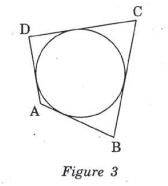14. Draw a line segment AB of length 7 cm. Using ruler and compass, find a point P on AB such that15. Find the perimeter of the shaded region in Figure 4, If ABCD is a square of side 14 cm and APB and CPD are semicircles.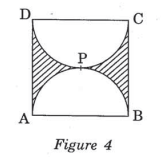16. Two cubes each of volume 27 cm3 are joined end to end to form a solid. Find the surface area of resulting cuboid.

### OR

A cone of height 20 cm and radius of base 5 cm is made up of modelling clay. A child reshapes it in the form of a sphere. Find the diameter of the sphere.

17. Find the value of y for which the distance between the points A (3, -1) and B(11, y) is 10 units.

18. A ticket is drawn at random from a bag containing ticket of numbered from 1 to 40. Find the probability that the selected ticket has a number which is a multiple of 5.

### Section C

Question number 19 to 28 carry 3 marks each.

19. Find the roots of the following quadratic equation:20. Find an A.P. Whose fourth term is 9 and the sum of its sixth term and thirteenth term is 40.

21. In Figure 5, a triangle PQR is drawn to circumscribe a circle of radius 6 cm such that the segment OT and TR into which QR is divided by the point of contact T, are of length 12 cm and 9 cm respectively. If the area ofPQR = 189 cm2, then find the length of sides PQ and PR.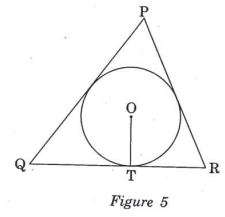22. Draw a pair of tangents to a circle of radius 3 cm, which are inclined to each other at an angle of 60°.

### OR

Draw a right triangle in which the sides (other than hypotenuse) are of lengths 4 cm and 3 cm. Then construct another triangle. Whose sides aretimes, the corresponding sides of the given triangle.

23. A chord of a circle of radius 14 cm subtends an angle of 120° at the centre. Find the area of the corresponding minor segment of the circle.24. An open metal bucket is in the shape of a frustum of a cone of height 21 cm with radii of the lower and upper ends as 10 cm and 20 cm respectively. Find the cost of milk which can completely fill the bucket at Rs. Per little.25. Point P (x, 4) lies on the line segment joining the points A (-5, 8) and B (4, -10). Find the ratio in which point p divides the line segment AB. Also find the value of x.

26. Find the area of the quadrilateral ABCD, whose vertices are A(-3, -1), B(-2, -4), C(4, -1) and D (3, 4)

### OR

Find the area of the triangle of formed by joining the mid-points of the sides of the triangle whose vertices are A (2, 1), B (4, 3) and C (2, 5).

27. From the top of a vertical tower, the angle of depression of two cars, in the same straight line with the base of the tower, at an instant are founded to be 45° and 60°. If the cars are 100 m apart of the dice, whose product is 12.

28. Two dice are rolled once. Find the probability of getting such number on the two dice, whose product is 12.

### OR

A box contains 80 discs which are numbered from 1 to 80. If one disc is drawn at random the box, find the probability that it bears a perfect square number.

### Section D

Question numbers 29 to 34 carry 4 marks each.

29. prove that the tangent at any point of a circle is perpendicular to the radius through the point of contact.

30. The first and the last terms of an A.P. are 8 and 350 respectively. If its common difference is 9, how many terms are there and what is their sum?

OR

How many multiples of 4 lie between 10 and 250? Also, find their sum.

31. A train travels 180 km at a uniform speed. If the speed had been 9 km/hour more, it would have taken 1 hour less for the same journey. Find the speed of the train.

OR

Find the roots of the equation32. In figure 6, three circle each of radius 3.5 cm are drawn in the such a way that each of the touches the other two. Find the area enclosed. Between these three circle (shaded region).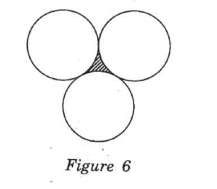33. Water is flowing at the rate of 15km/hour through a pipe of diameter 14 cm into a cuboidal pond which is 50m long and 44m wide. In what time will the level of water in the pond rise by 21 cm?

34. The angle of elevation of the top of a vertical tower from a point on the ground is 60°. From another point 10 m vertically above the first, its angle of elevation is 30°. Find the height of the tower.

These are questions only. To view and download complete question paper with solution install myCBSEguide App from google play store or login to our student dashboard.

## CBSE Question Paper 2011 class 10 Mathematics

Download class 10 Mathematics question paper with solution from best CBSE App the myCBSEguide. CBSE class 10 Mathematics paper 2011 in PDF format with solution will help you to understand the latest question paper pattern and marking scheme of the CBSE board examination. You will get to know the difficulty level of the question paper.

## Previous Year Question Paper for class 10 in PDF

question papers 2019, 2018, 2017, 2016, 2015, 2014, 2013, 2012, 2011, 2010, 2009, 2008, 2007, 2006, 2005 and so on for all the subjects are available under this download link. Practicing real question paper certainly helps students to get confidence and improve performance in weak areas.

To download CBSE Question Paper class 10 Mathematics, Science, Social Science, English Communicative, English Language and Literature, Hindi Course A, Hindi Course B, and Other subjects; do check myCBSEguide app or website. myCBSEguide provides sample papers with solution, test papers for chapter-wise practice, NCERT solutions, NCERT Exemplar solutions, quick revision notes for ready reference, CBSE guess papers and CBSE important question papers. Sample Paper all are made available through the best app for CBSE students and myCBSEguide website.Test Generator

Create question paper PDF and online tests with your own name & logo in minutes.myCBSEguide

Question Bank, Mock Tests, Exam Papers, NCERT Solutions, Sample Papers, Notes